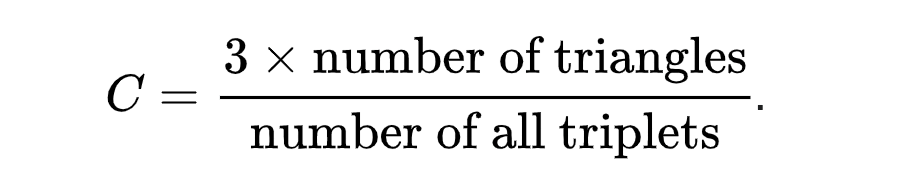# Change Nickname

Current Nickname:
Search
v2.x
v2.x

### `algo(hyperANF)`

HyperANF is an approximate algorithm that calculates the average distance of nodes, which by defination is the sum of distances (length of the shortest path) of all node pairs divided by the total number of node pairs. It is implemented on Ultipa Graph in a real-time (synchronous) fashion, and gives approximate result rather than exact value.

The theoretical value of HyperANF of nodes in a graph is: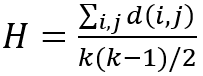Where d is the distance of two nodes and k is the total number of nodes. Any node pair having no path in between does not participate in the calcultation. The result of this forluma stays no less than 1, whether the graph is connected or not.

HyperANF is short for Approximating the Neighborhood Function of Very Large Graphs on a Budget, a fairly recent graph algorithm invented by Italian scientists Paolo Boldi, Marco Rosa, Sebastiano Vigna in their 2011 paper. HyperANF is a breakthrough improvement over ANF in terms of speed and scalability. It leverages modern-day multi-core X86 architecture for maximal parallelization, without which, the ANF computing may take much longer time (exponentially longer) and consume much more underpinning computing resources.

In a social network, the average distance of nodes represents how easily one can make contact with any other person in the network. It aligns with the famous Six Degrees of Separation that all people on average are six, or fewer, social connections away from each other. Researches show that the average distance of real-world social networks like Facebook and Twitter is 4 ~ 5, which suggests 3 ~ 4 connections between any two network users.

Configuration items for HyperANF operation:

Item Data Type Specification Description
`<loop_num>` int >0 The number of loops or iterations (the accuracy of calculation increases with the loop number until the loop number reaches the distance of the longest node pair)
`<register_num>` int [4, 30] The exponent empoloyed in a power function used by HyperLogLog for cardinality counting

Calculation results:

Item Data Type Range
the approximate average distance float >=1

Validity of `write_back()`:

Not supported.

The below screenshot shows how HyperANF is invoked inside Ultipa Manager: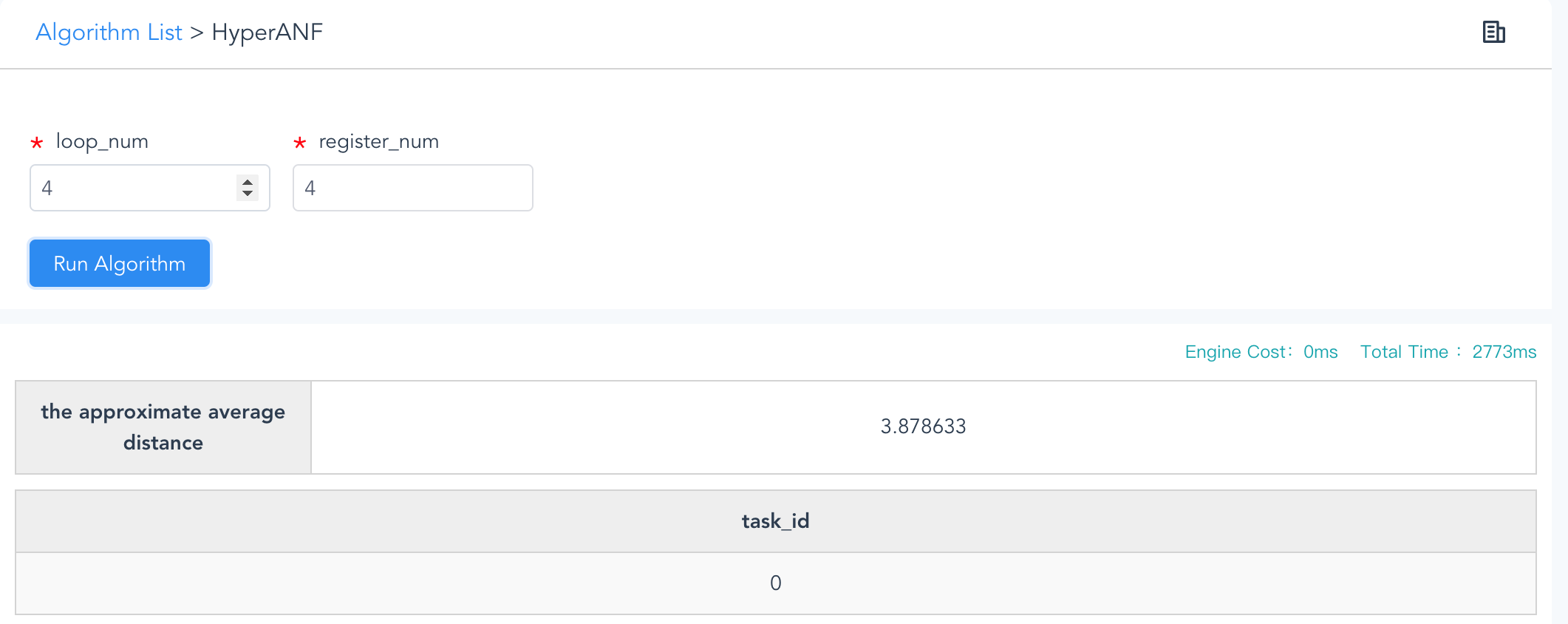HyperANF (Real-time with Small-to-Mid-Size Graphset)

### `algo(knn)`

K-nearest neighbors (K-NN) algorithm calculates the value of a particular property of a node, called label, based on the labels of its k-nearest neighbors. It works by finding out nodes that have top-k Cosine Similarity to the subject node, and selecting their most frequent label as the label of the subject node: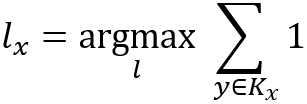Where, K is the top-k-node set of the subject node x, the labels of all the top-k-nodes are grouped by value and counted, the label with the highest count is assigned to node x. In case multiple labels are found with the highest count, select the one whose node has higher similarity.

`For details on the calculation of Cosine Similarity please refer to the chapter of Similarity.`

K-NN captures both ideas of LPA (Label Propagation Algorithm) and similarity (sometimes called distance, such as squared Euclidean distance, or cosine similarity, proximity, or closeness), and is implemented in machine learning algorithms, either supervised or not, to solve both classification (selecting the most frequent label) and regression (averaging the label values) problems.

Configuration items for K-NN operation:

Item Data Type Specification Description
`<node_id>` int Ultipa ID The node to calculate
`<node_property_names>` []string comma (,) separated, at least two properties (numeric type) The node properties (must be LTE first) to be used for similarity calculation
`<top_k>` int >0 The number of nearest neighbors
`<target_property_name>` string node property The node property/label to be calculated

Calculation results:

Item Data Type Range
the selected label / /
the count of the selected label int >0
the cosine similarity of each top-k node float [0, 1]

Validity of `write_back()`:

Not supported.

Example: Given node_id 12 and properties 'age' and 'salary', to predicate 'grade' and top 10 most similar nodes:

``````algo(knn).params({
node_id: 12,
node_property_names: ["age", "salary"],
top_k: 10,
})
``````

### `algo(k_core)`

A k-core of a graph G is a maximal connected subgraph of G in which all nodes have a degree (excluding the contribution by self-loop edges) of at least k. Equivalently, it may be one or several connected components of G formed by a pruning process that recursively deletes all nodes that have an up-to-date degree of less than k until no more node to be deleted. A non-empty k-core means that G has degeneracy at least k, namely, the degeneracy of G is the largest k for which G has a k-core.

K-core is widely adopted to find the densest part of a network given a density level. For instance, identifying groups of people in a social network that are more closely intra-group coupled than people outside the groups; targeting groups with frequent transactions.

Configuration items for K-Core operation:

Item Data Type Specification Description
`<k>` int / The minimum number of degrees of nodes in the resulting subgraphs

Calculation results:

Item Data Type Range
the id of nodes of the k-core subrgraph int Ultipa ID

Validity of `write_back()`:

Approach Destination
To database /
To disk file RPC port

The screenshot from below shows what happens on Ultipa Manager when invoking K-core with k=5.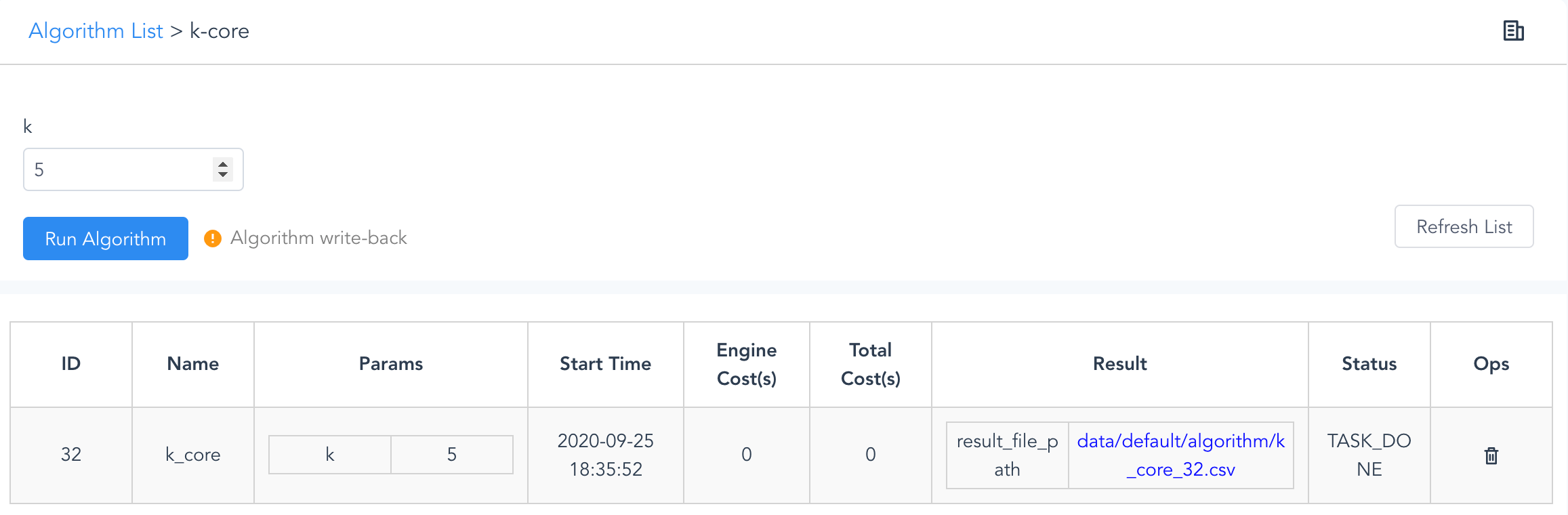K-Core (Ultipa Manager)

### `algo(mst)`

Minimum Spanning Tree, shortened as MST, is to connect all nodes within the graph to form a sub-graph that has minimum aggregated weight, and the sub-graph doesn't contain any circles (therefore it's a tree, essentially) - by leveraging a certain edge property as weight.

Theoratically, multiple solutions of MST may be found for a graph, and the MST algorithm by Ultipa Graph calculates and returns one of these solutions with a given node to start as root.

For cases of disconnected graph, a root is needed for each connected component to generate a complete MST; the MST starts from the first root provided, and fully grows in the first component before moving onto the next root; the isolcated nodes are not a legal part of the MST since they have no edges and do not contribute in the total weight.

Note: for a connected graph, the number of edges of its MST is equal to the graph's number of nodes minus 1. It can be inferred that for any graph, the number of edges of its MST plus the number of its connected components plus the number of its isolated nodes is equal to the graph's number of nodes.

Configuration items for MST operation:

Item Data Type Specification Description
`<ids>` []int Ultipa ID The starting nodes/roots of connected components; later queued nodes from a duplicated component are not valid
`<edge_property_name>` string edge property (numeric type) The edge property (must be LTE first) to be used as weight factor
`<limit>` int >0; -1 The maximum number of results to return; -1: return all the results

Calculation results:

Item Data Type Range
the number of edges of the MST int Ultipa ID
the id of edges int Ultipa ID

Validity of `write_back()`:

Approach Destination
To database /
To disk file RPC port

Example: Starting from nodes id=[1,5,12], and using edge property 'rank' to calculate its MST:

``````algo(mst).params({
ids: [1,5,12],
edge_property_name: "distance",
limit: -1
})
``````

The below diagram shows how MST is executed inside Ultipa Manager: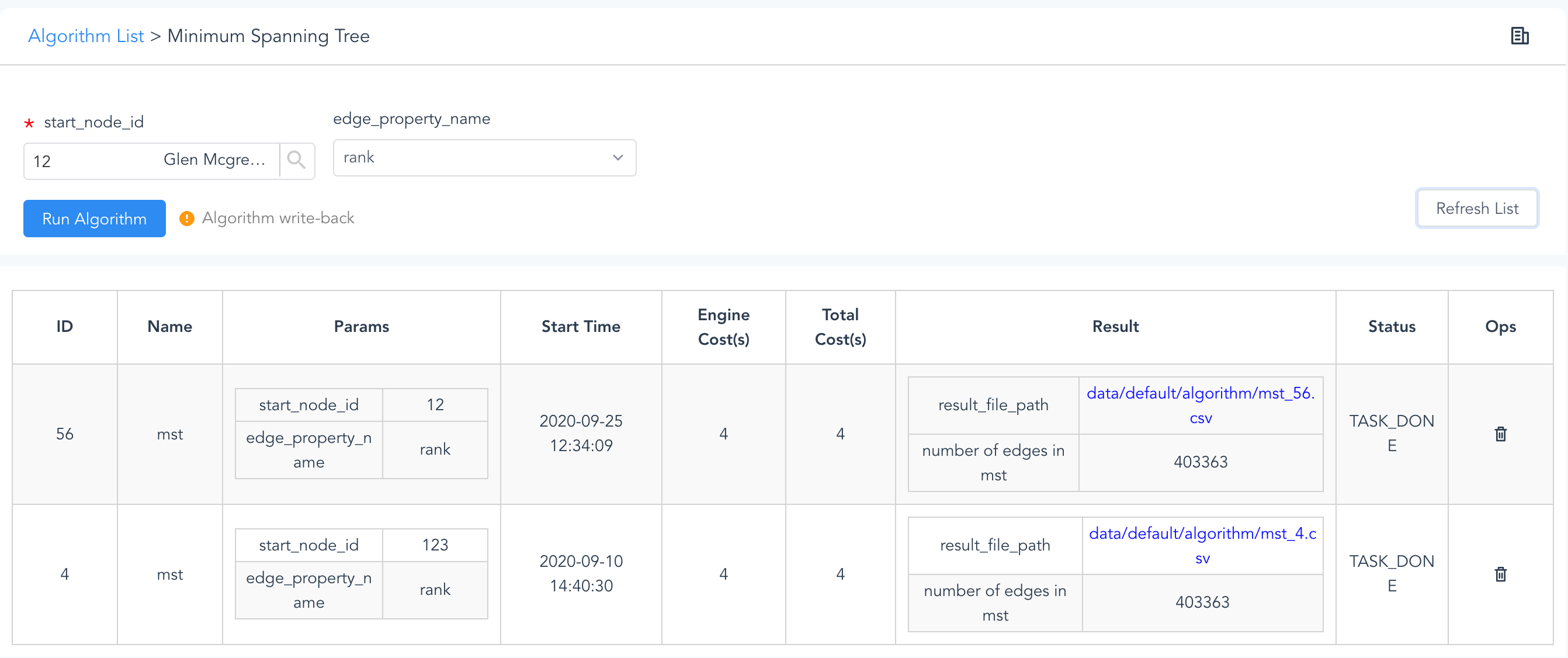MST (Ultipa Manager)

### `algo(k_means)`

K-means clustering partitions nodes into k clusters by assigning each node to the cluster with the nearest mean (cluster's geometric center) to that node. This is done through an iterative process, at the beginning of which a number of k nodes are selected as the initial means; during each iteration all the nodes are re-assigned to the nearest mean, after which each mean is re-calculated using the nodes assigned to it. The iteration stops when each mean converges individually, or when the iteration limit has been reached.

The nearest mean found for a subject node in each iteration using Euclidean distance is: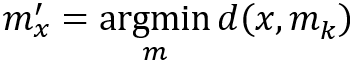Where, d is the Euclidean distance to each mean from the subject node x, and a mean with the minimum distance is the one that node x will be assinged to.

Below is the variant of the above formula when employing Cosine Similarity to define 'nearest', in which a mean with the maximum similarity s is selected: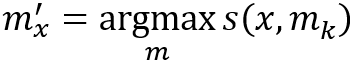Here are some tips for the initial mean selection: different initial means might generate different clustering solutions; a duplicated initail mean will produce an empty cluster.

Configuration items for K-Means operation:

Item Data Type Specification Description
`<k>` int >0 The number of clusters
`<start_ids>` []int Ultipa ID The k nodes to be used as the initial means of k clusters; do not use nodes with duplicated mean values
`<node_property_names>` []string node property (numeric type) A list of node properties (must be LTE first) to be used for the distance (similarity) calculation, at least one property for Euclidean distance and two for Cosine Similarity
`<distance_type>` int 1; 2 1: Euclidean distance
2: Cosine Similarity
`<loop_num>` int >0 The number of loops or iterations, keep it small if possible

Calculation results:

Item Data Type Range
the number of nodes of each cluster int >=0
cluster id of each node int >=0

Validity of `write_back()`:

Approach Destination
To database /
To disk file RPC port

Example 1: By using cosine similarity, the 'age' and 'salary' of node, loop 5 times to calculate K-Means, starting with [1, 4, 13]:

``````algo(k_means).params({
k: 3,
start_ids: [1, 4, 13],
node_property_names: ["age", "salary"],
distance_type: 2,
loop_num: 5
})
``````

### `algo(clustering_coefficient)`

Clustering Coefficient by Ultipa Graph calculates the local clustering coefficient of a node, which measures the completeness of the maximal subgraph formed by the neighbors of the subject node. According to the definition of complete graph, by which for each pair of nodes in a graph there is edge(s) connecting both nodes, this algorithm calculates the ratio of number of neighbor pairs with in-between edge(s) to the total number of neighbor pairs. See the formula: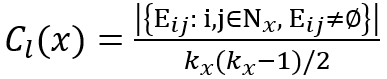Where, E stands for the nonempty set of edges between neighbor i and j of the subject node x, and |{E}| is the total number of E; k stands for the number of neighbors of the subject node hence k*(k-1)/2 is the number of neighbor pairs.

The local clustering coefficient by Ultipa Graph is considered a real-time one. It can be applied to reflect how well one's friends know each other, and the local clustering coefficient of a real-world social network is usually higher than that of a network with randomly created edges between nodes.

Configuration item for local Clustering Coefficient operation:

Item Data Type Specification Description
`<node_id>` int Ultipa ID The node to calculate

Calculation results:

Item Data Type Range
the local clustering coefficient float [0, 1]

Validity of `write_back()`:

Not supported.

Example: Calculate local clustering coefficient of node id=12:

``````algo(clustering_coefficient).params({ node_id: 12 })
``````

Here is the screenshot of running local clustering coefficient inside Ultipa Manager: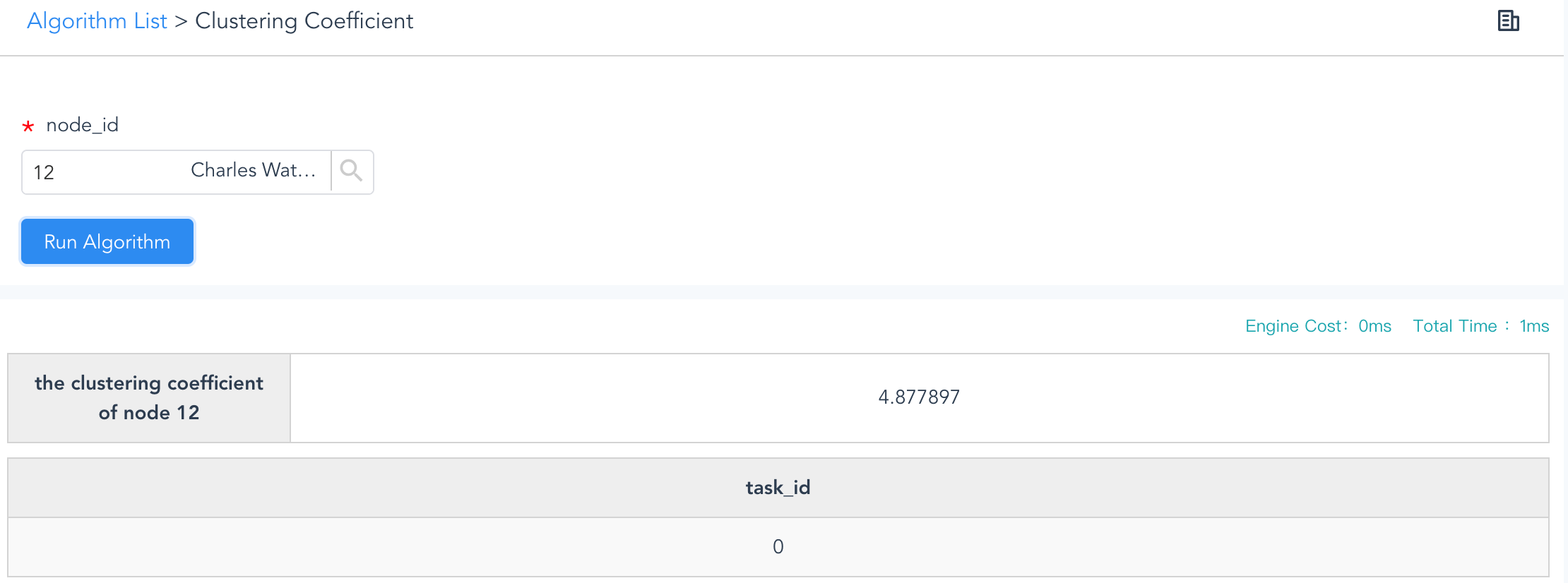Clustering Coefficient (Ultipa Manager)

To validate the accuracy of the clustering coefficient, you can first get the subject node's 1-hop neighbors, and run an `autoNet()` of depth=1 for all these neighbors with a very large limit, so that you can verify all links exist amongst these neighbors.

As a side-note, another type of clustering coefficient, which is global clustering coefficient that will be offered soon, is calculated using the following formula: